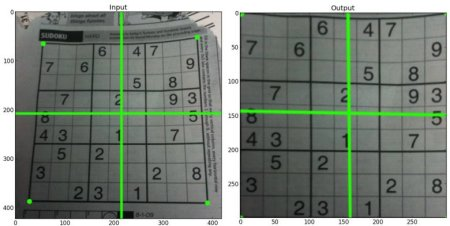# 图像透视变换¶

## 概要¶

keywords 投影 perspective-projection 图像变形

## 什么是透视变换¶Geometric Transformations of Imagesimport cv2
import matplotlib.pyplot as plt
import numpy as np

rows,cols,ch = img.shape
# 左图中画面中的点的坐标 四个
pts1 = np.float32([[56,65],[368,52],[28,387],[389,390]])
# 变换到新图片中，四个点对应的新的坐标 一一对应
pts2 = np.float32([[0,0],[300,0],[0,300],[300,300]])

# 生成变换矩阵
M = cv2.getPerspectiveTransform(pts1,pts2)
# 进行透视变换
dst = cv2.warpPerspective(img,M,(300,300))

plt.subplot(121),plt.imshow(img),plt.title('Input')
plt.subplot(122),plt.imshow(dst),plt.title('Output')
plt.show()


## 透视变换的应用¶

[[[ 922  632]]

[[ 659 2694]]

[[3794 2630]]

[[3362  857]]]


# 因为之前膨胀了很多次，所以四边形区域需要向内收缩而且本身就有白色边缘
margin=40
pts1 = np.float32([[921+margin, 632+margin], [659+margin, 2695-margin], [3795-margin, 2630-margin], [3362-margin, 856+margin]])


A4纸的宽高比在 1.4 ： 1, 所以定义新图片的四个顶点。

pts2 = np.float32([[0,0], [0, 1000], [1400, 1000], [1400, 0]])

M = cv2.getPerspectiveTransform(pts1,pts2)
dst = cv2.warpPerspective(gray,M,(1400,1000))